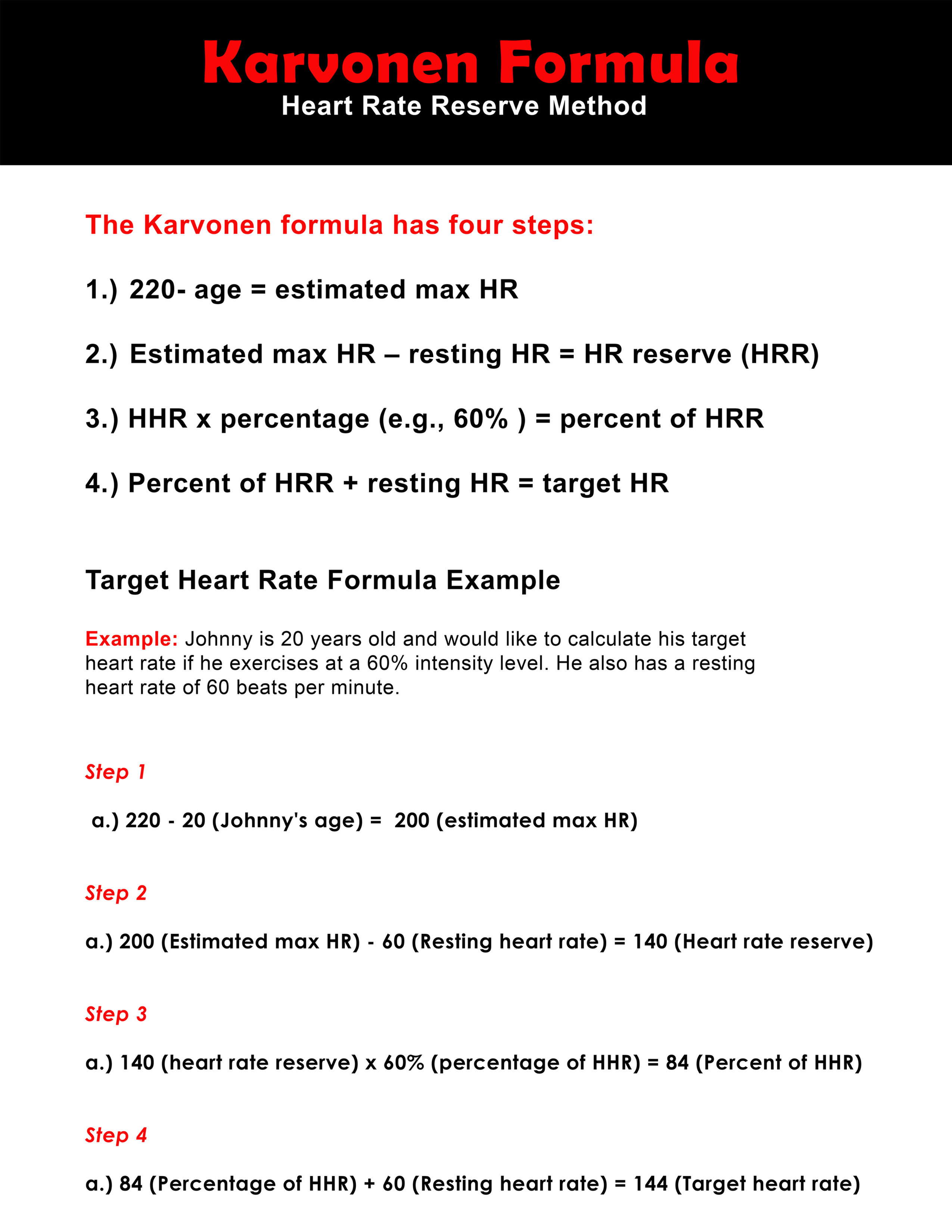# Karvonen Formula

The Karvonen formula or percentage heart rate reserve (%HHR) method is the most accurate way to find your target heart rate. This method considers your age, resting heart rate and maximum heart rate.

When using the Karvonen formula, the resting heart rate is subtracted from the maximal heart rate. The percentage HHR is calculated using the percentage of exercise intensity. A percentage of HHR is added to the clients resting HR to determine the target exercise HR.

There are 4 steps to using the Karvonen formula:

1. 220- age = estimated max HR

2. Estimated max HR – resting HR = HR reserve (HRR)

3. HHR x percentage (e.g., 70% ) = percent of HRR

4. Percent of HRR + resting HR = target HR

Here’s an example of this formula in action:

For example, Johnny is 20yr old with a resting heart rate of 60. He wants to know his training heart rate for the intensity level 60%.

Step 1:

220 - 20 (Johnny’s Age) = 195 (Estimated max HR)

Step 2:

200 (Estimated max HR) – 60 (Resting heart rate) = 140 (Heart rate reserve)

Step 3:

140 (heart rate reserve) x 60% (percentage of HHR) =84 (Percent of HHR)

Step 4:

84 (Percentage of HHR) + 60 (Resting heart rate) = 144 (Target heart rate)

Johnny’s Target heart rate would be 144 using the method above.

If you need a quick way to find your target heart rate use the Karvonen formula calculator

Below is a mini poster you can print to remember this formula or put on your wall.